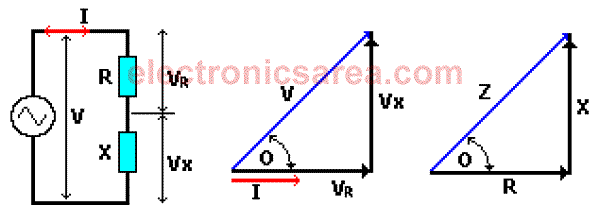# Power Factor Definition

Power Factor (PF) is the ratio between the energy that is converted into work and the electrical energy consumed in a circuit or device. In other words, power factor is the ratio between the total voltage applied to a circuit and the voltage in the resistive circuit part. PF is also:

• – Cosine of the angle (cos0) between the vectors of real power and apparent power.
• – Cosine of the angle (cos0) between impedance and resistance vectors.

The AC power, consumed by a circuit with resistive elements (resistors) and reactive elements (capacitors and / or inductors) can be obtained with the following formulas: P = I x V x PF or P = IRMS VRMS x PFNotes:

• RMS means Root Mean Square
• PF = Power Factor

See the circuit above and the corresponding phasor diagram. Although the diagram represents an inductive value, the procedure is valid in general.

The current (I) and voltage (VR) are in phase in the resistor R. The power (real power) dissipated in an impedance Z = (R + jX), is due only to resistance. Then: P = I x VR

From the phasor diagram: VR = V cos(0).
Combining the last two formulas we get: P = I x Vcos(0)

Comparing this equation with the expression: P = I x V x power factor, we conclude that: Power factor = cos (0). Where 0 is the phase angle of the impedance, or in other words, 0 is the angle between the voltage and current in the circuit.

Then PF = Cos(0) = VR/V = R/|Z|

Where: |Z| means the absolute value of Z (Z value is always positive, regardless of the sign)

The angle value will always be between:

• 0 °: Where V and I are in phase (fully resistive circuit). Cos (0) = 1, then PF = 1
• 90: When V and I are 90 ° out of phase (fully reactive circuit). Cos (0) = 0, PF = 0

We seek to have a power factor as high as possible, where cos(0) tends to “1” (most resistive possible).

•
•
•
•
•
•
•
•
•
•
•
•
•
•
•
•
•
•